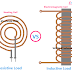The main difference between Resistive Load and Inductive Load is resistive load converts the electrical energy into heat whereas the inductive load converts the electrical energy into magnetic energy. There are ao many differences between inductive load and resistive are available. Let's know all of them.

Some examples of resistive load are electric heater, filament bulbs, etc. Resistive loads are very constant in nature. They only oppose the flow of current and do not make any changes in the voltage and current.

Resistive load generally produces heat when an electrical supply provided to it. Resistive loads are very simple in construction also. They only have a coil of conductive wires. The main function of the resistive load is to produce heat and it depends upon the length and cross-sectional of the wire. Also, it depends upon the duration of the current flow and its magnitude.

Some major properties of a resistive load are,

1. They do not make any phase difference between voltage and current.
2. The resistive load cannot store electrical energy, in fact, they waste electrical power in form of heat.
3. Resistive load always shows the same characteristics for both AC and DC supply.
4. Resistive load always causes a unity power factor.
5. The resistive load cannot produce reverse power to the circuit.

Some examples of Inductive Loads are Electric Motor, Induction cookers, etc. Inductive loads are those which not constant in nature. Their characteristics change with changes in the nature of the power supply. In fact, they can change the nature of the power supply. Inductive loads are the more power-consuming or heavy load among others because they can drive the mechanical load also. Inductive load not constant in nature also, unlike a resistive load it can take any amount of current according to its applications.

Some major properties of an Inductive Load are,

1. The inductive load can make a phase difference between voltage and current.
2. Inductive load shows different properties for AC and DC. In fact, its properties depend upon the frequency also.
3. The inductive load can store electrical energy in form of a magnetic field for a very short time.
4. Inductive load always causes a lagging power factor.
5. The inductive load can produce reverse power to the circuit.

Difference between Resistive Load and Inductive LoadReviewed by Author on June 09, 2021 Rating: 5